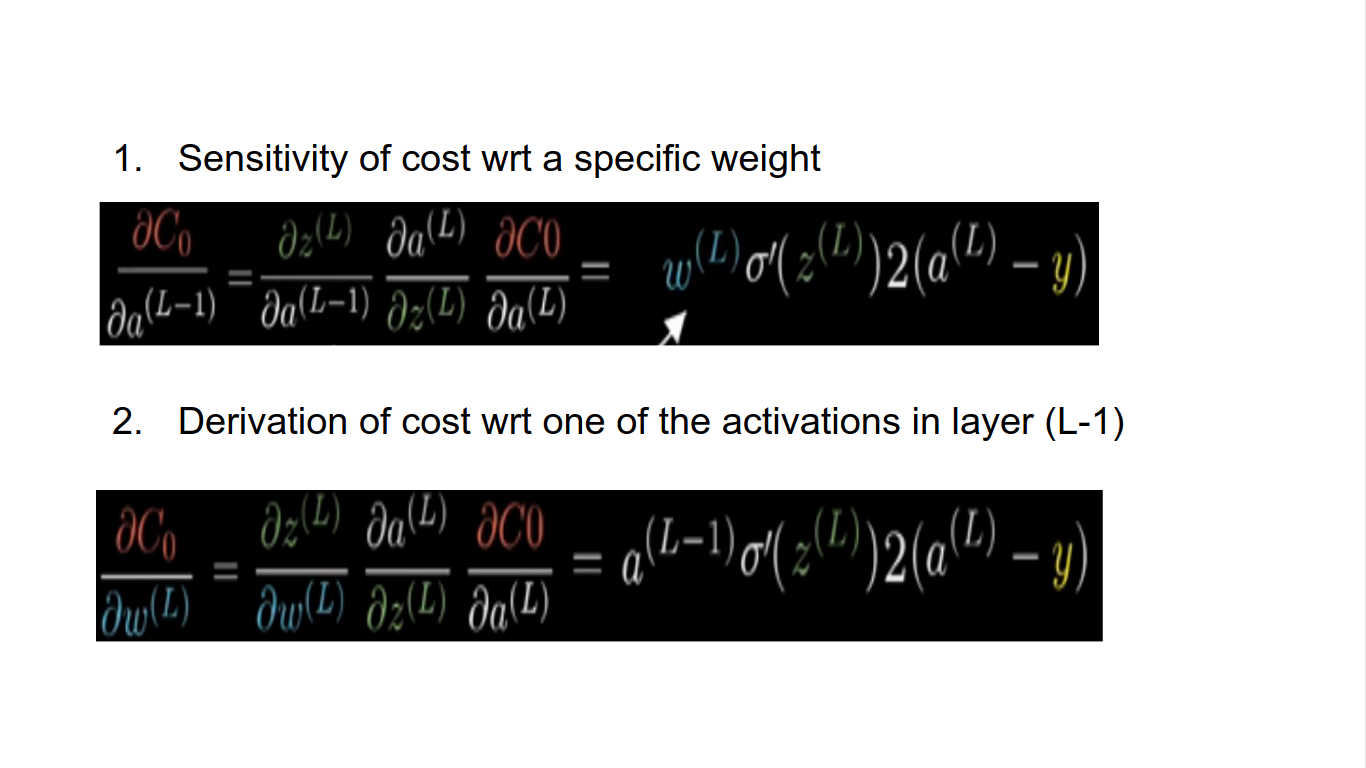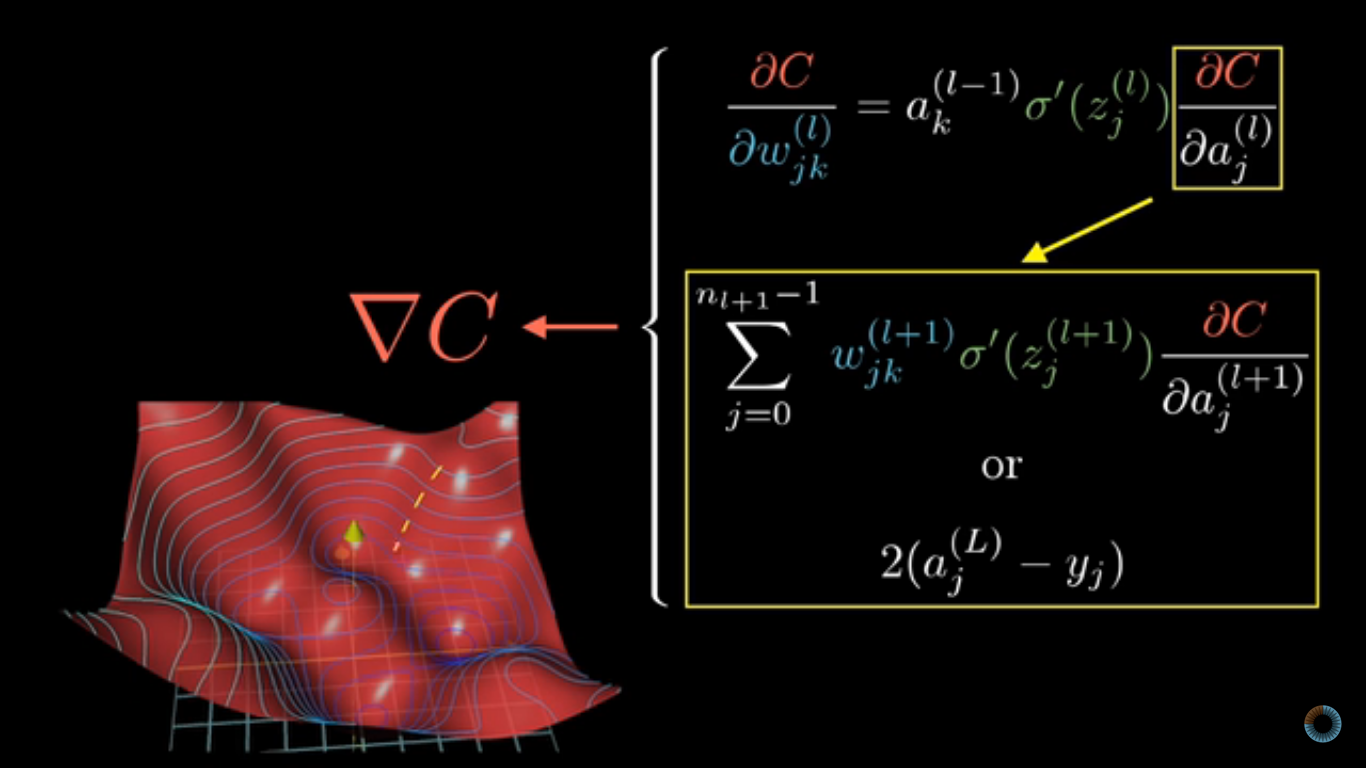# Deep Neural Network (DNN) in a Brief

An introduction to deep neural networks

### Neural Network (NN):

It is a series of algorithms that endeavors to recognize underlying relationships in a set of data through a process that mimics the way the human brain operates.

### Examples and Applications of NN:

1. Convolutional neural network => Good for image recognition
2. Long Short-Term memory network => Good for Speech Recognition

### Neuron:

1. It is a mathematical function that models the functioning of a biological neuron.
2. It computes the weighted average of its input and passes the sum through a non-linear function called the activation function (such as the sigmoid).

### Training an Artificial neural network:

1. The process of repeated nudging an input of a function by some multiple of the negative gradient is called Gradient Descent.
2. When there are one or more inputs, you can use Gradient descent for optimizing the values of the coefficients by iteratively minimizing the error of the model on your training data.
3. A learning rate is used as a scale factor and the coefficients are updated in the direction towards minimizing the error.
4. This process is repeated until a minimum sum squared error is achieved or no further improvement is possible.

### Backward Propagation:

1. It is an algorithm for supervised learning of an ANN using Gradient Descent.
2. Given an ANN and an error function it calculates the gradient of the error function w.r.t. the NN weights.
3. Here the partial computations of the gradient from one layer are reused in the computation of the gradient for the previous layer.

### Backpropagation Calculation:

• The chain rule expressions give us the derivatives that determine each component in the gradient that helps to minimize the cost of the network by repeatedly stepping downhill.Tags:

Categories:

Updated: